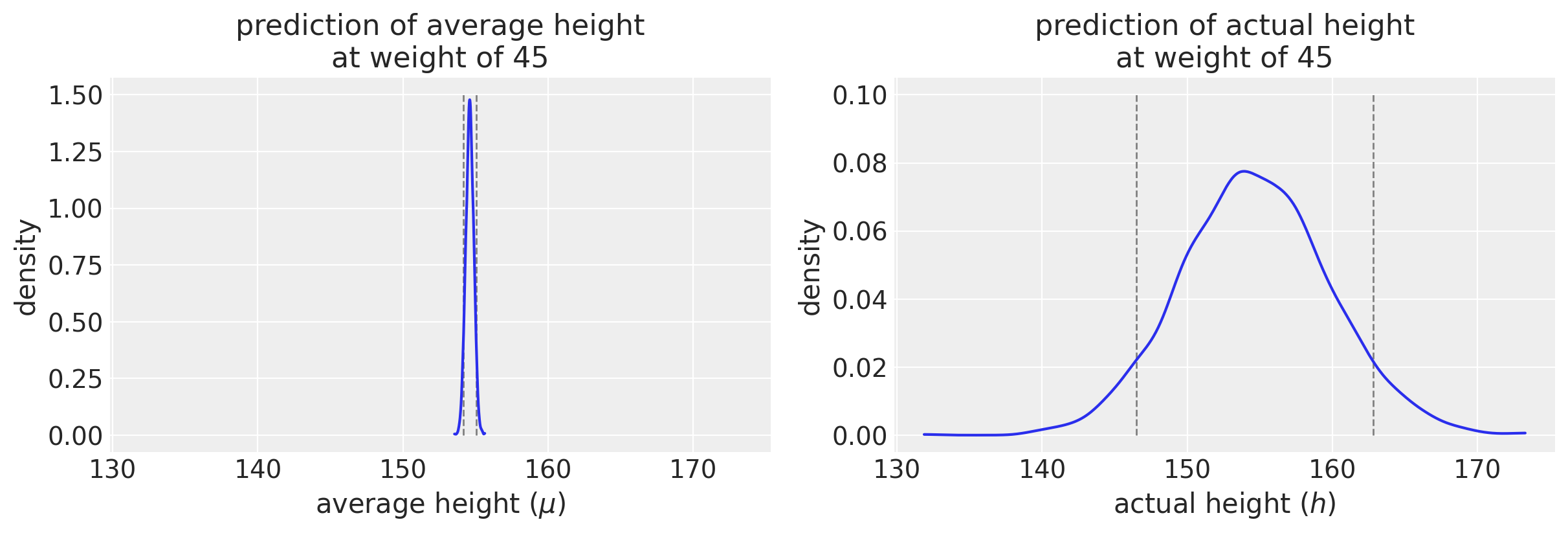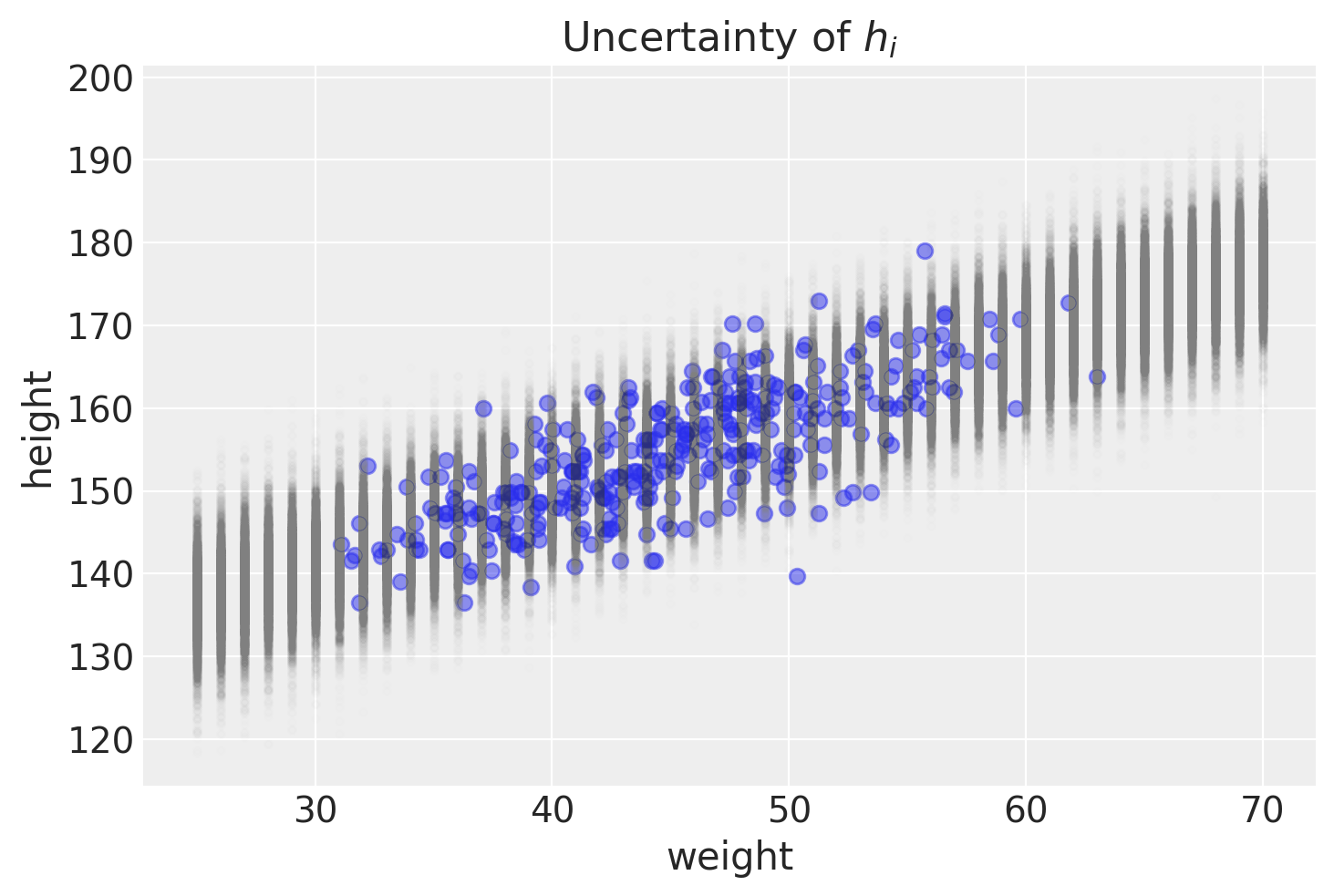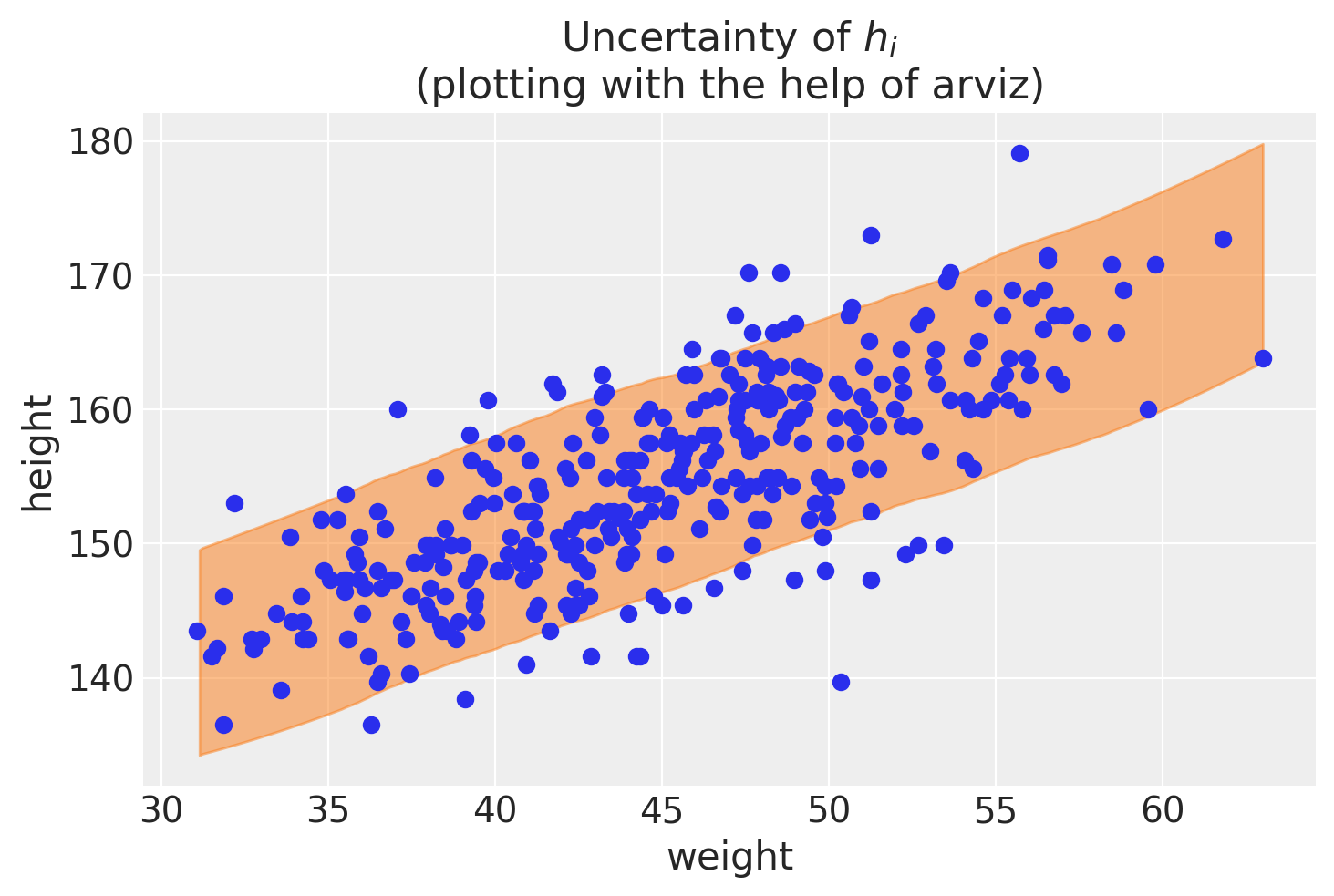# PyMC linear regression part 4: predicting actual height

At last, we have come to the end. This is the final post in a series of linear regression posts using PyMC3, from my reading of Statistical Rethinking. Part 1 set up the problem and explored the package’s objects. Part 2 interpreted the posterior distribution. Part 3 predicted average height which has its own uncertainty. Here, we’ll predict actual height which avoids the over-confidence that comes from predicting average height only.

Going deep into each step took some time, but it’s helped embed the concepts in my brain. As we’ve done before, we’ll start off duplicating code of the previous posts before we make the final predictions.

import arviz as az
import matplotlib.pyplot as plt
import numpy as np
import os
import pandas as pd
import pymc3 as pm
import scipy.stats as stats
import seaborn as sns

%load_ext nb_black
%config InlineBackend.figure_format = 'retina'
RANDOM_SEED = 8927
np.random.seed(RANDOM_SEED)
az.style.use("arviz-darkgrid")


Question

Ths question come’s from the winter 2020, week 2 homework.

The weights listed below were recorded in the !Kung census, but heights were not recorded for these individuals. Provide predicted heights and 89% compatibility intervals for each of these individuals. That is, fill in the table below, using model-based predictions.

Individual weight expected height 89% interval
1 45
2 40
3 65
4 31

Let’s quickly take a look at the data to get a handle on what we’re working with.

d = pd.read_csv("../data/a_input/Howell1.csv", sep=";", header=0)
d2 = d[d.age >= 18]  # filter to get only adults


# Setting up the variables

# Get the average weight as part of the model definition
xbar = d2.weight.mean()

with pm.Model() as heights_model:

# Priors are variables a, b, sigma
# using pm.Normal is a way to represent the stochastic relationship the left has to right side of equation
a = pm.Normal("a", mu=178, sd=20)
b = pm.Lognormal("b", mu=0, sd=1)
sigma = pm.Uniform("sigma", 0, 50)

# This is a linear model (not really a prior or likelihood?)
# Data included here (d2.weight, which is observed)
# Mu is deterministic, but a and b are stochastic
mu = a + b * (d2.weight - xbar)

# Likelihood is height variable, which is also observed (data included here, d2.height))
# Height is dependent on deterministic and stochastic variables
height = pm.Normal("height", mu=mu, sd=sigma, observed=d2.height)

# The next lines is doing the fitting and sampling all at once.
trace_m2 = pm.sample(1000, tune=1000, return_inferencedata=False, progressbar=False)

Auto-assigning NUTS sampler...
Initializing NUTS using jitter+adapt_diag...
Multiprocess sampling (4 chains in 4 jobs)
NUTS: [sigma, b, a]
Sampling 4 chains for 1_000 tune and 1_000 draw iterations (4_000 + 4_000 draws total) took 12 seconds.

trace_m2_df = pm.trace_to_dataframe(trace_m2)


The next set of code gives both the MAP as well as providing a sense of the variance of the data, which is more useful.

az.rcParams["stats.hdi_prob"] = 0.89  # sets default credible interval used by arviz
az.summary(trace_m2, round_to=3, kind="stats")

/Users/blacar/opt/anaconda3/envs/stats_rethinking/lib/python3.8/site-packages/arviz/data/io_pymc3.py:88: FutureWarning: Using from_pymc3 without the model will be deprecated in a future release. Not using the model will return less accurate and less useful results. Make sure you use the model argument or call from_pymc3 within a model context.
warnings.warn(

mean sd hdi_5.5% hdi_94.5%
a 154.600 0.275 154.178 155.055
b 0.903 0.043 0.835 0.970
sigma 5.103 0.195 4.789 5.404
# Covariance matrix
trace_m2_df.cov().round(3)

a b sigma
a 0.075 0.000 0.000
b 0.000 0.002 -0.000
sigma 0.000 -0.000 0.038

# Predictions for actual height

We return now to the original problem where we are seeking predictions for actual height. We will produce compatability intervals where we’d expect to see any observed height (note how the uncertainty region for $\mu_i$ as shown above is much narrower than where the points are.)

We will do something similar as in the previous post but incorporating $\sigma$.

$\text{height}_i$ ~ Normal($\mu_i, \sigma$)

The repo initially confused me, but I saw that the solution was straightforward after more exploring of functions. I’ll spare you most of this exploration, but will put some of my key insights here.

Function exploration
One key realization for me was seeing how the stats function for drawing from a normal distribution can have varied mean (loc) and standard deviation (scale) values. Previously, I had typically used it to get multiple samples from the same mean and standard deviation like this:

stats.norm.rvs(loc=10, scale=2, size=10)

array([ 7.09403309, 12.255019  ,  7.32435791, 10.26594505, 11.79233188,
12.09804331, 10.84097672, 14.51428249, 14.22362859,  9.25777341])


I hadn’t previously thought to use different mean and standard deviations for each draw. That is, loc and scale can be vectors. I’ll vary the vectors down below for each so that you can see what I mean in the resulting vector.

stats.norm.rvs(
loc=[1, 1, 1, 10, 10, 10, 20, 20, 20], scale=[0.5, 1, 2, 0.5, 1, 2, 0.5, 1, 2]
)

array([ 0.73038967,  0.37624878,  1.87080349,  9.87713139, 10.7586794 ,
9.36231624, 20.29745599, 19.36382859, 18.66087831])


This is when I had my a-ha moment about what to do next. Remember: the posterior distribution that pymc outputs produces a different mean and standard deviation in each line.

trace_m2_df.head()

a b sigma
0 154.030585 0.853788 4.989442
1 153.969973 0.861619 5.128790
2 155.111070 0.957769 5.067568
3 154.106523 0.838728 4.961068
4 154.328210 0.863311 5.160797

We don’t need to use a multivariate normal distribution function but we can instead use the regular (non-multivariate?) version of the normal distribution function. This implementation is more consistent with R code 4.63 which I copied/posted below taken from McElreath’s repo.

## R code 4.63
post <- extract.samples(m4.3)
weight.seq <- 25:70
sim.height <- sapply( weight.seq , function(weight)
rnorm(
n=nrow(post) ,
mean=post$a + post$b*( weight - xbar ) ,
sd=post$sigma ) ) height.PI <- apply( sim.height , 2 , PI , prob=0.89 )  ## Making predictions for actual height ($h_i$) at a single weight value When we input a weight, we can create a vector for height that results from the normal distribution that consists of the posterior’s parameters. This seems confusing at first, but let’s just look at one weight, 45. Note how the parameters within the stats.norm.rvs function mimic McElreath’s R code above. example_weight = 45 h_at_45 = stats.norm.rvs( loc=trace_m2_df["a"] + trace_m2_df["b"] * (example_weight - xbar), scale=trace_m2_df["sigma"], ) print(h_at_45) print("Length of vector: ", len(h_at_45))  [155.7948111 146.56221928 159.43749538 ... 155.33600385 149.83412841 151.31287874] Length of vector: 4000  We have a vector of values for which we can compute summary statistics, including getting a compatibility interval. # Get 89% compatibility interval az.hdi(np.array(h_at_45))  array([146.08867804, 162.48740096])  This seems repetitive to what we did for average height in the last post, but here we’ve incorporated sigma from our posterior distribution. Let’s repeat the code for average height and compare what we just did for actual height. # Vector for average height - note that sigma is not used! mu_at_45 = trace_m2_df["a"] + trace_m2_df["b"] * (45 - xbar)  f, (ax1, ax2) = plt.subplots(1, 2, figsize=(12, 4), sharex=True) az.plot_kde(mu_at_45, ax=ax1) ax1.set_title("prediction of average height\nat weight of 45") ax1.set_ylabel("density") ax1.set_xlabel("average height ($\mu$)") ax1.vlines( az.hdi(np.array(mu_at_45)), ymin=0, ymax=1.5, color="gray", linestyle="dashed", linewidth=1, ) az.plot_kde(h_at_45, ax=ax2) ax2.set_title("prediction of actual height\nat weight of 45") ax2.set_ylabel("density") ax2.set_xlabel("actual height ($h$)") ax2.vlines( az.hdi(np.array(example_draw)), ymin=0, ymax=0.1, color="gray", linestyle="dashed", linewidth=1, )  <matplotlib.collections.LineCollection at 0x7fe5ad6e2940>I am intentionally plotting the x-axis with the same axis limits so that you can appreciate the difference in uncertainty. (Note the difference in the y-axis scale.) We would expect that the probability distribution for average height would be much tighter than the one for actual height. The plots drive that point home visually. ## Making predictions for actual height ($h_i$) at a single weight value We’ll start off by generating a matrix that contains the posterior predictions for actual heights over a range of weights, between 25 and 70 kg. This is similar to what we did for average heights in the last post. # Input a range of weight values weight_seq = np.arange(25, 71) # This is generating a matrix where the predicted h values will be kept # Each weight value will be its own row h_pred = np.zeros((len(weight_seq), len(trace_m2_df))) # Fill out the matrix in this loop for i, w in enumerate(weight_seq): h_pred[i] = stats.norm.rvs( loc=trace_m2_df["a"] + trace_m2_df["b"] * (w - xbar), scale=trace_m2_df["sigma"] ) # Output the matrix in a dataframe for viewing df_h_pred = pd.DataFrame(h_pred, index=weight_seq) df_h_pred.index.name = "weight" df_h_pred.head()  0 1 2 3 4 5 6 7 8 9 ... 3990 3991 3992 3993 3994 3995 3996 3997 3998 3999 weight 25 146.819087 131.950216 129.793433 141.425616 134.363193 134.193045 138.625503 127.385217 135.971128 132.278403 ... 128.438620 128.187695 140.142773 136.868872 132.564979 140.662170 135.461373 129.179569 133.174905 139.440426 26 136.456664 139.880879 138.150449 144.660997 130.087085 133.466438 134.668864 130.054509 137.989162 144.520142 ... 140.724558 122.746829 141.763728 140.855240 138.828098 125.438146 139.518013 145.103823 144.708165 137.155993 27 133.427578 146.873131 148.040623 134.562287 143.913001 141.570235 138.758449 126.249154 139.976170 136.232402 ... 132.069314 142.616321 139.039138 144.439965 140.008615 132.783098 136.572733 131.534774 135.076601 145.095471 28 143.316194 130.229996 135.166804 140.417059 143.595620 146.727791 142.071108 135.161632 138.845736 140.713138 ... 131.457780 131.502991 140.776164 144.107353 150.544606 144.113096 133.674960 137.908407 131.267668 141.673441 29 142.093570 142.195453 145.842916 144.098927 146.044805 138.547015 148.580323 134.374695 139.463730 141.527401 ... 142.074329 145.405100 144.384839 131.004655 150.856133 139.352867 133.089377 139.672434 139.466480 133.085828 5 rows × 4000 columns To re-iterate, each row are a weight’s sampling of heights as dictated by the posterior distribution of parameters. We can illustrate that graphically. # Plotting the data as a scatter plot plt.scatter(d2["weight"], d2["height"], alpha=0.5) plt.plot(weight_seq, h_pred, "C0.", color="gray", alpha=0.005) plt.xlabel("weight") plt.ylabel("height") plt.title("Uncertainty of$h_i$")  Text(0.5, 1.0, 'Uncertainty of$h_i')PyMC method for predictions of actual height The above plot helps show the uncertainty for a range of weights after some step-by-step calculations. Not surprisingly, pymc and arviz can help us get to those steps more quickly. Here’s one way to do that. # Predict heights # set parameter to 200 to match the notebook example # could set to 400 to match the samples and nchains height_pred = pm.sample_posterior_predictive(trace_m2, 200, heights_model) height_pred  /Users/blacar/opt/anaconda3/envs/stats_rethinking/lib/python3.8/site-packages/pymc3/sampling.py:1687: UserWarning: samples parameter is smaller than nchains times ndraws, some draws and/or chains may not be represented in the returned posterior predictive sample warnings.warn( <div> <style> /* Turns off some styling */ progress { /* gets rid of default border in Firefox and Opera. */ border: none; /* Needs to be in here for Safari polyfill so background images work as expected. */ background-size: auto; } .progress-bar-interrupted, .progress-bar-interrupted::-webkit-progress-bar { background: #F44336; } </style> <progress value='200' class='' max='200' style='width:300px; height:20px; vertical-align: middle;'></progress> 100.00% [200/200 00:00<00:00] </div> {'height': array([[152.44015719, 143.8660879 , 139.40217284, ..., 156.3123799 , 162.99350218, 160.15982149], [161.48691291, 152.72929135, 138.60784035, ..., 162.5252433 , 167.12620516, 160.8162708 ], [154.18712134, 148.66960604, 140.74062707, ..., 165.56777632, 160.19434841, 157.95943753], ..., [160.39855129, 147.75720026, 140.01191018, ..., 159.5460152 , 153.48386574, 163.72311138], [149.698651 , 142.51656198, 145.54450618, ..., 167.74115779, 165.92839171, 164.0738555 ], [159.73732783, 153.56864439, 141.05003146, ..., 166.80582294, 161.04500287, 160.92227836]])}  f, ax1 = plt.subplots() # ax = az.plot_hdi(weight_seq, mu_pred.T) az.plot_hdi(d2["weight"], height_pred["height"], ax=ax1) ax1.scatter(d2["weight"], d2["height"]) ax1.set_xlabel("weight") ax1.set_ylabel("height") ax1.set_title("Uncertainty ofh_i$\n(plotting with the help of arviz)")  /Users/blacar/opt/anaconda3/envs/stats_rethinking/lib/python3.8/site-packages/arviz/stats/stats.py:493: FutureWarning: hdi currently interprets 2d data as (draw, shape) but this will change in a future release to (chain, draw) for coherence with other functions warnings.warn( Text(0.5, 1.0, 'Uncertainty of$h_i\$\n(plotting with the help of arviz)')# The answer to the problem

After all that, we come to the answer, which can be written in a few lines of code.

# Initialize the table
df_answer["individual"] = range(1, 5)
df_answer["weight"] = [45, 40, 65, 31]

# Fill out the table
for i, weight in enumerate(df_answer["weight"]):

draw = stats.norm.rvs(
loc=trace_m2_df["a"] + trace_m2_df["b"] * (weight - xbar),
scale=trace_m2_df["sigma"],
)

df_answer.loc[i, "expected_height"] = "{0:0.2f}".format(draw.mean())
# .at is for insertion of alist
# https://stackoverflow.com/questions/26483254/python-pandas-insert-list-into-a-cell
df_answer.at[i, "89perc_interval"] = "{0:0.2f}, {1:0.2f}".format(
az.hdi(draw), az.hdi(draw)
)

# Output the table

individual weight expected_height 89perc_interval
0 1 45 154.55 146.06, 162.41
1 2 40 150.22 141.98, 157.86
2 3 65 172.79 164.58, 180.99
3 4 31 141.96 133.63, 149.96

# Summary: A lesson about two kinds of uncertainty

In a series of posts, I took a deep dive into this problem. I initially had not appreciated the two kinds of uncertainty that this problem illustrates. It took me some time to absorb the concept. This was in the McElreath book.

Rethinking: Two kinds of uncertainty. In the procedure above, we encountered both uncertainty in parameter values and uncertainty in a sampling process. These are distinct concepts, even though they are processed much the same way and end up blended together in the posterior predictive simulation. The posterior distribution is a ranking of the relative plausibilities of every possible combination of parameter values. The distribution of simulated outcomes, like height, is instead a distribution that includes sampling variation from some process that generates Gaussian random variables. This sampling variation is still a model assumption. It’s no more or less objective than the posterior distribution. Both kinds of uncertainty matter, at least sometimes. But it’s important to keep them straight, because they depend upon different model assumptions. Furthermore, it’s possible to view the Gaussian likelihood as a purely epistemological assumption (a device for estimating the mean and variance of a variable), rather than an ontological assumption about what future data will look like. In that case, it may not make complete sense to simulate outcomes.

Appendix: Environment and system parameters

%watermark -n -u -v -iv -w

Last updated: Wed May 19 2021

Python implementation: CPython
Python version       : 3.8.6
IPython version      : 7.20.0

numpy     : 1.20.1
scipy     : 1.6.0
arviz     : 0.11.1
seaborn   : 0.11.1
json      : 2.0.9
pandas    : 1.2.1
matplotlib: 3.3.4
pymc3     : 3.11.0

Watermark: 2.1.0


Categories:

Updated: